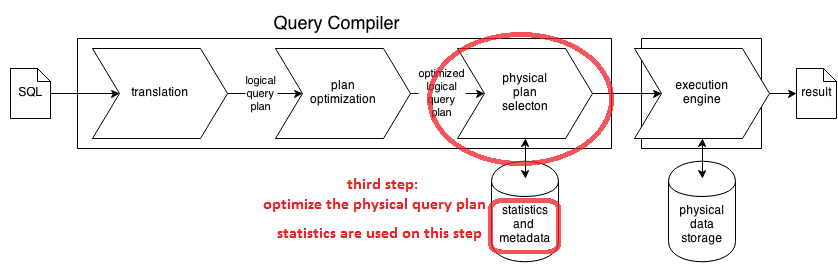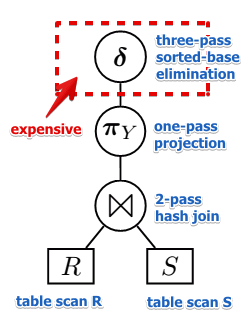# ML Wiki

## Physical Query Plan OptimizationTranslating SQL to RA expression is the first step in Query Processing Pipeline

• Input: Optimized Logical Query plan - expression in Extended Relational Algebra
• Output: Optimized Physical Query Plan - expression in Relational algebra with each node assigned some physical algorithm

## Cost-Based Plan Selection

We need to select the optimal plan based on

This is an Optimization Problem

## Greedy Algorithm

At each step we choose the operation with least local cost

Bottom-up approach:

• first assign physical operators to leaves
• then to parents of the leaves
• then to their parents
• and so on
• at each step choose an operator that gives the lowest cost
• for join operators use a gredy algorithm for join ordering

### Limitations

Doesn't take into account the properties of the output of an operator

Consider this example:

•• suppose optimized sort-merge join is not available - not enough memory
• so the Greedy algorithm decides to use hash-based join (recall that its output is not sorted)
• we could've taken slightly more expensive sort-merge join
• but instead of 3-pass duplicate elimination we'd just need one pass for $\delta$
• which would give us overall lower total cost

## Exercises

Main Article: Query Plan Selection Exercises